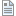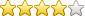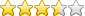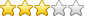Knowledgebase» Category: Charts  (Go back)

» Articles in this category:Consumption (kWh/kg/m3) ChartConsumption (kWh/kg/m3) The Consumption (kWh/kg/m3) bar chart shows the consumption for a meter (or set of meters) over a specific time period. For electricity consumption the bars are colour coded...Half Hourly kWh/kg/m3 Profile ChartHalf Hourly kWh/kg/m3 Profile Chart   The Half Hourly kWh/kg/m3 Profile line charts show a detailed view of the half hourly consumption data for meters with this level of data granularity ...Maximum Demand (kW) ChartMaximum Demand (kW) Chart Demand (kW) is a measure of the instantaneous draw of energy from the grid. A demand of 1kW for one hour will consume 1kWh of electricity. Demand is unique to electricity ...Half Hourly kW ProfileHalf Hourly kW Profile It is possible to analyse the half hourly demand in kW in the same way as the kWh consumption in Half Hourly kWh/kg/m3 Profile chart. Viewing the data in this format allows f...Average Power Factor ChartAverage Power Factor Chart The power factor of an electricity supply is a measure of how efficiently power is being consumed at site. For an ideal supply, the power factor would equal 1, which mean...Supply Utilisation ChartSupply Utilisation  Demand can also be measured in kVA, which takes into account the power factor of a site.  For a site with a power factor of one, the demand in kW will equal the demand...Summary by Time ChartSummary by Time Chart The Summary by Time pie chart summarises the consumption during the day/night and week/weekend for the selected meters/sites. It shows the total electricity and/or gas for eac...Summary by Area ChartSummary by Area Chart The Summary by Area pie chart summarises the consumption of each area of a site if there is an applicable site/meter hierarchy set up in ENMAT. It shows the total electricity ...Carbon Emissions ChartCarbon Emissions Chart The Carbon Emissions bar chart shows the kg CO2e (kg carbon dioxide equivalent) for a meter (or set of meters) over a specific time period. The kgCO2e is calculated from the ...Bill ForecastingBilling Forecast Chart The Billing Forecast chart calculates the cost of electricity and natural gas supplies based on the tariff information in ENMAT (see Energy Tariffs article). Default Cha...Production ChartProduction Chart The Production bar chart shows the consumption for a meter (or set of meters) over a specific time period in comparison to a Production metric. This is very useful for clients whos...